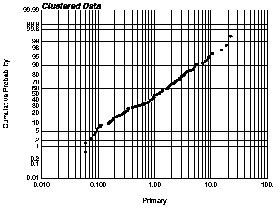GSLIB Help Page: PROBPLTDescription:
• probplt generates either a normal or a lognormal probability plot. This plot displays all the data values on a chart that illustrates the general distribution shape and the behavior of the extreme values. Checking for normality or lognormality is a secondary issue.Parameters:
• datafl: the data file in a simplified Geo-EAS format.
• icolvr and icolwt: column number for the variable and the weight. If icolwt less than or equal to 0, equal weighting is considered.
• tmin and tmax: all values strictly less than tmin and strictly greater than tmax are ignored.
• outfl: file for PostScript output.
• ilog: =0 then an arithmetic scaling of the variable scale is used, =1 then a logarithmic scaling (base 10) is used.
• pmin, pmax and pinc: minimum, maximum plotting limits, and increment for the variable axis.
• title: a 40-character title for the top of the plot. A short description of the program